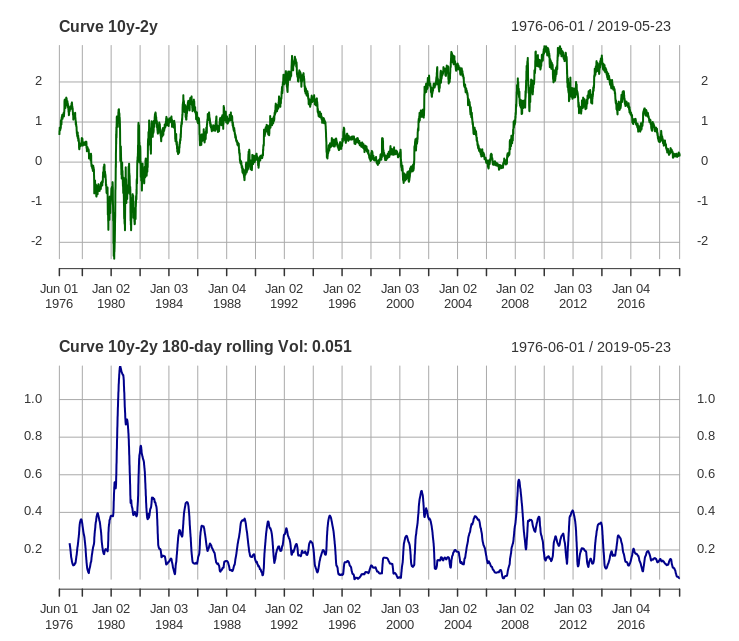Search
•John Hawver

# Curve Volatility at Lows

As I was reading my investment reports this weekend I noticed the yield curve (10y US Treasury yield minus the 2y US Treasury). It has been “stuck” between 18 and 22 basis points for quite some time. That lack of movement has wrung the volatility out of the curve.

Only 1996 and 2007 saw lower curve volatilities. It’s difficult to say if this is a predictor for any future move in the equity markets. I did an earlier post on the predictive power of the yield curve; curve volatility as a second order factor shouldn’t offer any more predictability.

Lack of curve volatility does make it more difficult for market makers to capture spread when they are hedging along the curve. It also tends to reduce the amount of hedging flow from other fixed income products like mortgages and convex products. This should mean continued reduced trading profits for banks and HFT market makers in US Treasury bonds.

I find it valuable as an investor to pay attention to things that don’t seem to quite make sense or aren’t following their historic norm. Negative yields in the EU bond markets are one of these things that continue to perplex me - seems a dangerous experiment.

Very low curve volatility I can understand, more or less caused by a Fed that has raised rates and used QE to constrain the bond market. However, given that very low levels of curve vol are often followed by spikes, it seems worthy of attention. That said, curve vol has been on a long grind down since 2009.

John#### Code to Replicate ####

# Setup

library('quantmod')

# Get data

tsy2y <- getSymbols('DGS2', src='FRED', return.class = "xts", auto.assign = FALSE)

tsy10y <- getSymbols('DGS10', src='FRED', return.class = "xts", auto.assign = FALSE)

# calc curve and curve vol

curve_10y_2y <- na.omit(tsy10y - tsy2y)

curve_vol <- na.omit(rollapply(curve_10y_2y, 180, sd))

# plot

par(mfrow=c(2,1))

plot(curve_10y_2y, main = 'Curve 10y-2y', col = 'darkgreen')

plot(curve_vol, main = paste0('Curve 10y-2y 180-day rolling Vol: ', round(tail(curve_vol, 1), 3)), col = 'darkblue')

# min values

curve_vol[which(curve_vol == min(curve_vol, na.rm = T))]

curve_vol[which(curve_vol <= as.numeric(tail(curve_vol, 1)))]

See All